Function Repository Resource:

# CurveAberrancy

Compute the aberrancy of a plane curve

Contributed by: Jan Mangaldan
 ResourceFunction["CurveAberrancy"][c,t] computes the aberrancy of the plane curve c parametrized by variable t.

## Details

The aberrancy of a plane curve at a given point is defined as the tangent of the angle between the normal through that point, and the axis of the osculating parabola through that point.

## Examples

### Basic Examples (3)

Compute the aberrancy for a curve:

 In:=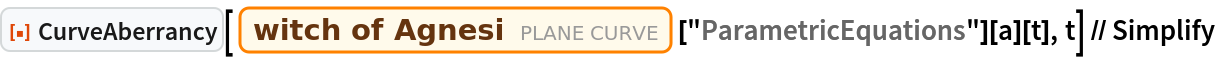Out=Compute the aberrancy for several curves:

 In:=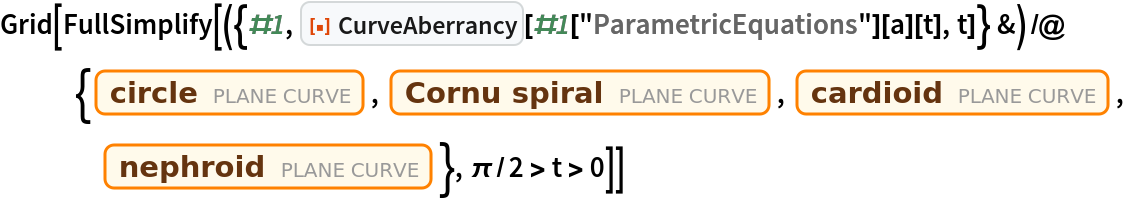Out=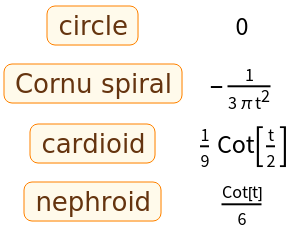Compute the aberrancy of a curve in polar coordinates:

 In:=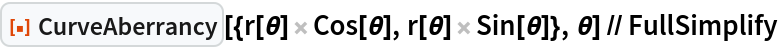Out=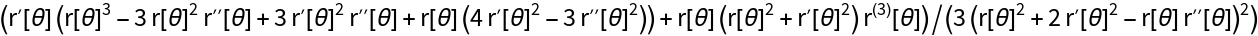### Properties and Relations (2)

The aberrancy of a curve involves third derivatives:

 In:=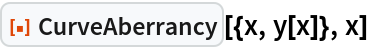Out=Aberrancy is related to the curvature and tangential angle:

 In:=Out=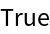## Version History

• 1.0.0 – 25 January 2021